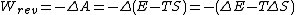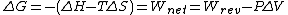# free energy

(redirected from Free energy change)
Also found in: Dictionary, Thesaurus, Medical.
Related to Free energy change: enthalpy, entropy

or

## Gibbs free energy,

quantity derived from the relationships between heat and work studied in thermodynamicsthermodynamics,
branch of science concerned with the nature of heat and its conversion to mechanical, electric, and chemical energy. Historically, it grew out of efforts to construct more efficient heat engines—devices for extracting useful work from expanding hot gases.
and used as a measure of the relative stability of a physical or chemical system, i.e., the tendency of the system to react or change. If the change in free energy, ΔG, is negative, the transformation of the system will occur spontaneously, since transitions in which the energy decreases are favored, whereas those in which it increases (ΔG positive) are not. The change in free energy for a given process at a particular temperature depends on three factors, as seen from the equation ΔG = ΔHTΔS, where ΔH is the change in the enthalpyenthalpy
, measure of the heat content of a chemical or physical system; it is a quantity derived from the heat and work relations studied in thermodynamics. As a system changes from one state to another the enthalpy change, ΔH,
of the system, T is the temperature in degrees Kelvin, and ΔS is the change in entropyentropy
, quantity specifying the amount of disorder or randomness in a system bearing energy or information. Originally defined in thermodynamics in terms of heat and temperature, entropy indicates the degree to which a given quantity of thermal energy is available for doing
. A negative value of the enthalpy change indicates a decrease in the heat content of the system and contributes to a favorable value of the free energy; a positive entropy change indicates a decrease in the orderliness of the system and also contributes to a favorable value of the free energy, since a system tends to go from more ordered to less ordered states. It may happen that the change in enthalpy for the reaction is favorable but that of the entropy is unfavorable, or vice versa; in such a case the temperature is the deciding factor since it determines how much weight is given to the entropy change. For example, in the transition of liquid water to ice, the enthalpy change is favorable because heat is released in the process but the entropy change is unfavorable because the transition is to the more ordered, crystalline state. Below a temperature of 32°F; (273°K;) the enthalpy term, ΔH, is larger and the process is spontaneous, but at higher temperatures the entropy term, TΔS, predominates, and the transition does not occur. Although the free energy indicates whether or not a given reaction will occur, it gives no information about the speed of such a reaction. The reaction of hydrogen with oxygen to form water has a favorable, negative, free energy, but the reaction rate is so slow that without the presence of a catalyst it is not observable. Scientists use tables listing the standard free energy, ΔG°, of various compounds; the standard free energy is the change in free energy when one mole of the compound is formed at 25°C; and 1 atmospheric pressure.

## Free energy

A term in thermodynamics which in different treatments may designate either of two functions defined in terms of the internal energy E or enthalpy H, and the temperature-entropy product TS.

The function (ETS) is the Helmholtz free energy and is the function ordinarily meant by free energy in European references. The Gibbs free energy is the function (HTS). For the Lewis and Randall school of American chemical thermodynamics, this is the function meant by the free energy F. To avoid confusion with the symbol F as applied elsewhere to the Helmholtz free energy, the symbol G has also been used. Another development was the introduction of the name free enthalpy, with symbol G, for the Gibbs function. See Work function (thermodynamics)

For a closed system (no transfer of matter across its boundaries), the work which can be done

(1)in a reversible isothermal process is given by the series shown in Eq. (1). For these conditions, TΔS represents the heat given up to the surroundings. Should the process be exothermal, TΔS < 0, then actual work done on the surroundings is less than the decrease in the internal energy of the system. The quantity (ΔE - TΔS) can then be thought of as a change in free energy, that is, as that part of the internal energy change which can be converted into work under the specified conditions. This then is the origin of the name free energy. Such an interpretation of thermodynamic quantities can be misleading, however; for the case in which TΔS is positive, Eq. (1) shows that the decrease in “free” energy is greater than the decrease in internal energy.

For constant temperature and pressure in a reversible process the decrease in the Gibbs function G for the system again corresponds to a free-energy change in the above sense, since it is equal to the work which can be done by the closed system other than that associated with its change in volume ΔV under the given constant pressure P. The relations shown in Eq. (2) can be formed since ΔH = ΔE + PΔV.

(2)Each of these free-energy functions is an extensive property of the state of the thermodynamic system. For a specified change in state, both ΔA and ΔG are independent of the path by which the change is accomplished. Only changes in these functions can be measured, not values for a single state.

The thermodynamic criteria for reversibility, irreversibility, and equilibrium for processes in closed systems at constant temperature and pressure are expressed naturally in terms of the function G. For any infinitesimal process at constant temperature and pressure, -dG ≥ δwnet. If δwnet is never negative, that is, if the surroundings do no net work on the system, then the change dG must be negative or zero. For a reversible differential process, -dG > δwnet; for an irreversible process, -dG > δwnet. The free energy G thus decreases to a minimum value characteristic of the equilibrium state at the given temperature and pressure. At equilibrium, dG = 0 for any differential process taking place, for example, an infinitesimal change in the degree of completion of a chemical reaction. A parallel role is played by the work function A for conditions of constant temperature and volume. Because temperature and pressure constitute more convenient working variables than temperature and volume, it is the Gibbs free energy which is the more commonly used in thermodynamics. See Entropy, Thermodynamic principles

McGraw-Hill Concise Encyclopedia of Physics. © 2002 by The McGraw-Hill Companies, Inc.
The following article is from The Great Soviet Encyclopedia (1979). It might be outdated or ideologically biased.

## Free Energy

a term used to refer to the Gibbs function and to the Helmholtz function, both of which are thermodynamic potentials. In Soviet usage, the term “free energy” is used primarily for the Helmholtz function.

The Helmholtz free energy, also called the work function, is defined as the difference between the internal energy U of a thermodynamic system and the product of the system’s entropy S and temperature T. The quantity ST, which is subtracted from the internal energy when the free energy is found, is sometimes called the bound energy.

## free energy

[¦frē ′en·ər·jē]
(thermodynamics)
The internal energy of a system minus the product of its temperature and its entropy. Also known as Helmholtz free energy; Helmholtz function; Helmholtz potential; thermodynamic potential at constant volume; work function.
McGraw-Hill Dictionary of Scientific & Technical Terms, 6E, Copyright © 2003 by The McGraw-Hill Companies, Inc.
References in periodicals archive ?
The data in Table 3 show that the standard Gibbs free energy change values for reactions at the methane production phase are all less than zero, signifying that all of the reactions occur spontaneously from left to right under standard conditions.
In the hydrolysis and acidification phases, the standard Gibbs free energy change [DELTA][G.sup.[theta]] < 0, and the reactions happen spontaneously; therefore, it is an entropy increasing process.
Table 1: Standard Gibbs free energy change when using glucose as fermentation substrate and bacteria for hydrolysis, acid production, and fermentation.
According to Table 2, the standard Gibbs free energy changes for most of the reactions at the hydrogen and acetic acid production phases are greater than zero, indicating that most of the reactions do not take place spontaneously from left to right under standard conditions.
[Delta][F.sub.t] = free energy change for the formation of critical nucleus due to non-ideality, J/k mol.
Systems Proton donor Proton acceptor Symmetric Asymmetric 1-Octanol O-Chloroaniline 3494 3399 O-Methoxyaniline 3485 3393 O-Methylaniline 3479 3394 P-Chloroaniline 3481 3396 P-Methylaniline 3469 3387 P-Methoxyaniline 3459 3382 Table 2: Formation constant and free energy change of Ortho and Para substitution of aniline dissolved in 1-Octanol in inert solvent C[Cl.sub.4] at 309 k.
The formation constant and free energy changes values for ortho and Para subsistent of anilines with 1-octanol systems in the inert solvent C[Cl.sub.4] are given in Table 2.
Interfacial energy, which is used to calculate the free energy change for nucleation, is determined by surface tension measurement.
Figure 1 shows the free energy change calculated for environmental pressure of 0 and -1 MPa as a function of bubble radius at different saturation pressure of 0.1, 0.2, 0.5, and 1.0 MPa.
As the pressure difference between saturation pressure and environmental pressure is increased, the critical free energy change is decreased resulting in increased rate of nucleation.

Site: Follow: Share:
Open / Close